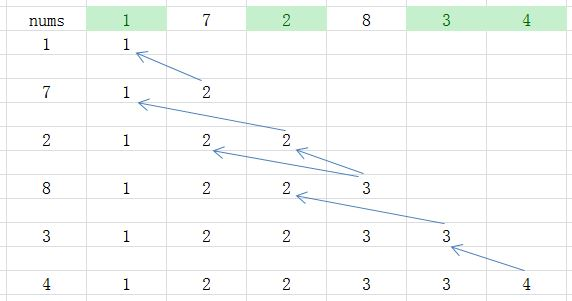# 动态规划

## 动态规划的本质，是对问题状态的定义和状态转移方程的定义。

dynamic programming is a method for solving a complex problem by breaking it down into a collection of simpler subproblems.

## 什么是状态的定义？

$F_k$为：以数列中第$k$项结尾的最长递增子序列的长度.

$F_1, ..., F_N$中的最大值.

## 什么是状态转移方程？

$F_k$为：以数列中第$k$项结尾的最长递增子序列的长度.

$A$为题中数列，状态转移方程为：

$F_1 = 1$（根据状态定义导出边界情况）

$F_k = max(F_{i} + 1 | A_{k} > A_{i}, i \in (1, ..., k-1)), (k > 1)$def lengthOfLIS(nums):
#Edge condition
if not nums:
return 0
dp = [1 for i in xrange(len(nums))]
for k in xrange(len(nums)):
for i in xrange(k):
# compare all F_i with F_k for i <ｋ
if nums[i] < nums[k]:
if dp[i] + 1 > dp[k]:
dp[k] = dp[i] + 1
return max(dp)

lengthOfLIS([1, 7, 2, 8, 3, 4])